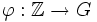# Tour:Equivalence of definitions of cyclic group

PREVIOUS: Cyclic group| UP: Introduction four (beginners)| NEXT: Every nontrivial subgroup of the group of integers is cyclic on its smallest element
General instructions for the tour | Pedagogical notes for the tour | Pedagogical notes for this part
WHAT YOU NEED TO DO:
• Read, and thoroughly understand, the equivalence of the two definitions of cyclic group. (We'll see better formulations of the proof later, after introducing homomorphisms and quotients)

## The definitions that we have to prove as equivalent

### Definition in terms of modular arithmetic

A group is said to be cyclic (sometimes, monogenic or monogenous) if it is either isomorphic to the group of integers or to the group of integers modulo n for some positive integer$n$.

### Definition in terms of generating sets

A group is termed cyclic (sometimes, monogenic or monogenous) if it has a generating set of size 1.

## Proof of equivalence

### From modular arithmetic to generating sets

This is direct:$\mathbb{Z}$ is generated by the element$1 \in \mathbb{Z}$, and$\mathbb{Z}/n\mathbb{Z}$ is generated by the element 1.

### From generating sets to modular arithmetic

Given: A group$G$ with a generating set$\{ g \}$

To prove:$G$ is isomorphic either to$\mathbb{Z}$ (the group of integers) or to$\mathbb{Z}/n\mathbb{Z}$ (the group of integers modulo n)

Proof: We consider two cases.

Case 1:$g$ has finite order. Thus, there exists a minimal positive integer$n$ such that$g^n$ is the identity element. Consider now the map$\varphi: \mathbb{Z}/n\mathbb{Z} \to G$ that sends$a$ to the element$g^a$. We want to prove that$\varphi$ is an isomorphism.

We first show that$\varphi(a + b) = \varphi(a)\varphi(b)$. For this, observe that if$a$ and$b$ add up to less than$n$ as integers, then$g^{a+b} = g^ag^b$ by definition. If the sum of$a$ and$b$ as integers is at least$n$, then$\varphi(a + b) = g^{a+b-n} =g^ag^bg^{-n} = g^ag^b$ (since$g^{-n}$ is the identity element).

Similarly,$\varphi(0) = g^0 = e$ by definition, and$\varphi(-a) = \varphi(a)^{-1}$, again because$g^n = e$.

Surjectivity: Since$g$ generates$G$, every element of$G$ can be written as a power of$g$, say$g^m$ for some integer$m$. Writing$m = nq + r$ where$q,r$ are integers and$r \in \{ 0,1,2,\dots,n-1 \}$, we get that$g^m = g^r = \varphi(r)$. Thus,$\varphi$ is surjective.

Injectivity: Finally, if$\varphi(a) = \varphi(b)$ with$a < b$ both in$\{ 0,1,2,\dots,n-1 \}$, then$g^{b - a} = e$, contradicting the assumption that$g$ has order$n$.

Thus,$\varphi$ is an isomorphism of groups.

Case 2:$g$ does not have finite order. In that case, consider the map$\varphi:\mathbb{Z} \to G$ that sends$n$ to$g^n$.

Clearly, by definition,$\varphi(a + b) = \varphi(a)\varphi(b)$,$\varphi(-a) = \varphi(a)^{-1}$, and$\varphi(0) = e$.

Surjectivity: Since$g$ generates$G$, every element of$G$ can be written as$g^n$ for some integer$n$.

Injectivity: If$\varphi(a) = \varphi(b)$ for$a < b$, then$g^{b - a} = e$, contradicting the assumption that$g$ does not have finite order.